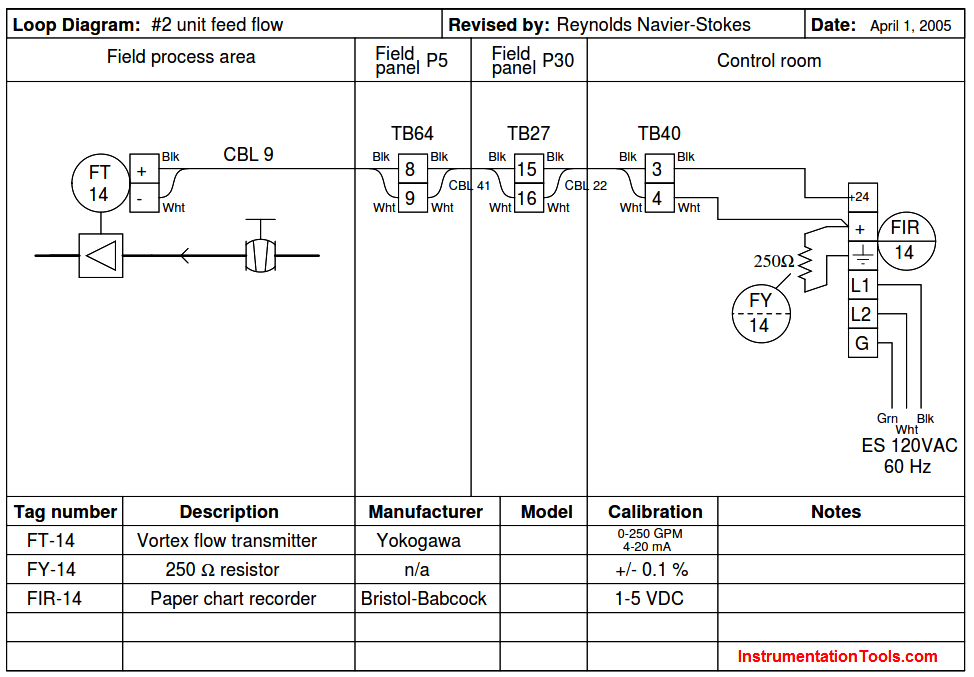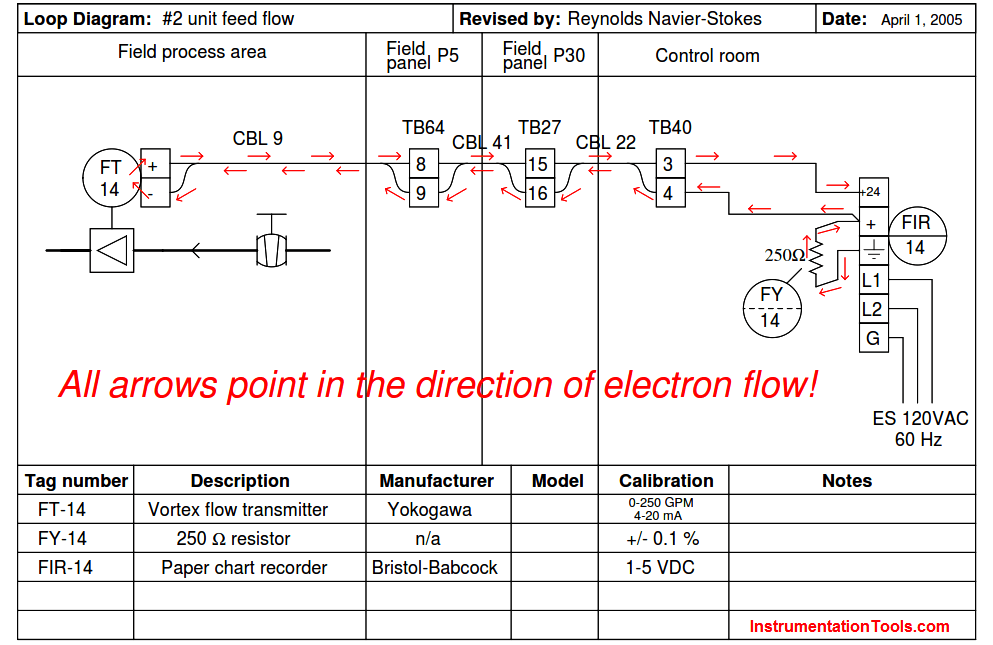# Determine Voltage Drops at Respective Flow Rates in Loop Diagram

In Flow Control Loop, Trace the path of current in the loop diagram signal wiring, then determine voltage drops at the respective flow rates. Examine the following loop diagram:

## Loop DiagramTrace the path of current in the signal wiring, then determine the following voltage drops at the respective ﬂow rates. Assume a power supply voltage of exactly 24 volts DC:

Voltage across FY-14 resistor = __________ ; Flow rate = 100 GPM

Voltage between terminals TB40-3 and TB40-4 = __________ ; Flow rate = 200 GPM

Voltage across FT-14 transmitter terminals = __________ ; Flow rate = 175 GPM

Voltage between terminals TB64-8 and TB27-15 = __________ ; Flow rate = 200 GPM

#### Solution:Voltage across FY-14 resistor = 2.6 volts ; Flow rate = 100 GPM

Voltage between terminals TB40-3 and TB40-4 = 19.8 volts ; Flow rate = 200 GPM

Credits: Tony R. Kuphaldt

Be the first to get exclusive content straight to your email.
We promise not to spam you. You can unsubscribe at any time.

### 5 thoughts on “Determine Voltage Drops at Respective Flow Rates in Loop Diagram”

1.How voltage across fy-14 is 2.6 volts at 100gpm can your ellaborate please???
My mail id engtarique451@gmail.com or whatsapp no. +96660330861

2.From the diagram, the flow transmitter range is 0 to 250 gpm

Now Calculate the loop current at 100 gpm, it is 10.4 mA

Calculate Voltage, V = I.R = 10.4mA x 250 = 2.6 v

You can use this online calculator: https://instrumentationtools.com/instrumentation-calculator/

3.Voltage across FY-14 resistor = __________ ;
Voltage between terminals TB40-3 and TB40-4 = __________ ;
Voltage across FT-14 transmitter terminals = __________ ;
Voltage between terminals TB64-8 and TB27-15 = __________ ;
if 80 GMP. thank you.
I need a sample.

4.Voltage across FY-14 resistor = 2.6 Volts ; Flow rate = 100 GPM
Voltage between terminals TB40-3 and TB40-4 = 24 volts ; Flow rate = 200 GPM
Voltage across FT-14 transmitter terminals = 24 volts ; Flow rate = 175 GPM
Voltage between terminals TB64-8 and TB27-15 = 24 volts ; Flow rate = 200 GPM

Why is the voltage between terminals TB40-3 and TB40-4 = 19.8 volts ; Flow rate = 200 GPM? It should be 24 volts because the analog output and inputs is 4 to 24 mA and constant voltage of 24 voltage because parallel connection from the PS to FT

5.